# Team:UCSF/Modeling2Modeling: Conjugation

The primary goal of the modeling portion for the CRISPRi conjugation project is to create a model that will help us identify the behavior of our transferable targeting system and identify critical parameters, given our assumptions. We used this model to check whether our CRISPRi conjugation system would have the desired behavior under biologically relevant parameters.

This system is designed to be transferred from cell to cell, targeting a specific gene of interest within the community. We model the activity of three strains of E. coli, an engineered donor strain containing a conjugative plasmid with genes coding for dCas9 and a gRNA, a recipient strain containing the RFP gene (target gene) in its genome, and upon successful conjugation, a transconjugate strain that no longer expresses RFP as well as the community's total RFP concentration.

Our objective is to simulate this community over time to see if our engineered system causes RFP levels to decrease.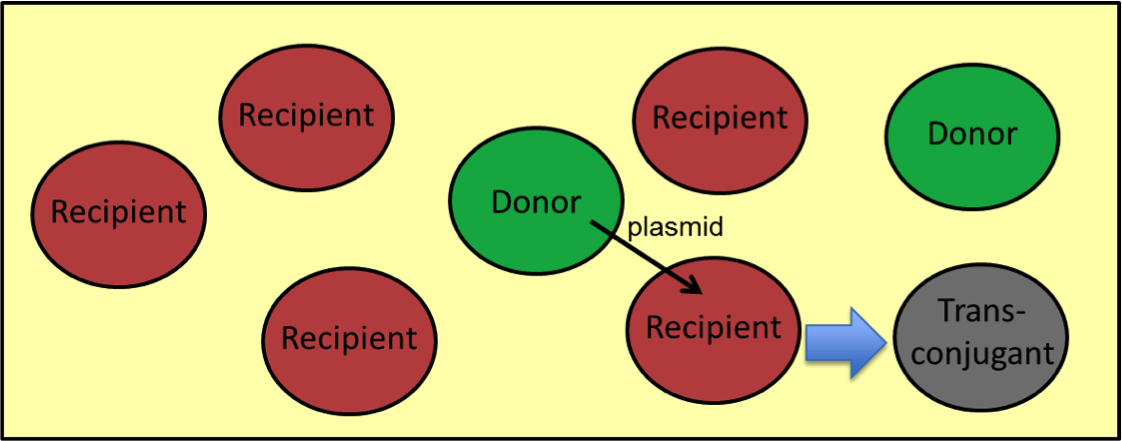ASSUMPTIONS: While creating the model for our system, we made four assumptions in order to simplify some of the aspects of the model. Many similar assumptions have been made in the literature.

1) RFP, dCas9, and gRNA are produced and degraded at a constant rate;
2) conjugation rate is linearly dependent on the concentration of donor and recipient cells (mass action);
3) CRISPR expression efficiency in a cell is 100%;
4) The growth rates of the three strains are equal.

EQUATIONS

Given these assumptions we have the following equations for the system:
Change in RFP levels over time: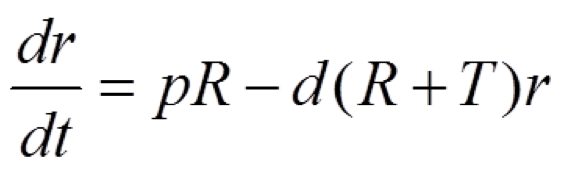where pR is the production term and d(R+T)r is the degradation term. The parameters in this equation are:

p – RFP production rate in a single cell
d – RFP degradation rate in a single cell

And the variables in this equation are:

r – concentration of RFP
t – time
R – number of recipient cells
T – number of transconjugant cells

In order to simulate the RFP levels, we need to model the population dynamics of our system. Thus, these equations have been to model the three strains present in this system:

Engineered Donor Cell levels: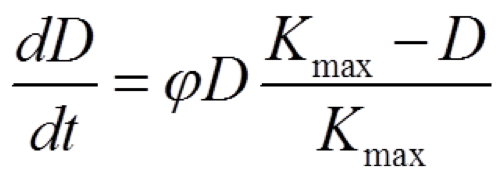RFP producing Recipient Cell levels: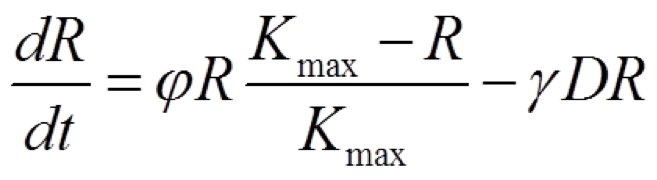Transconjugant Cell levels: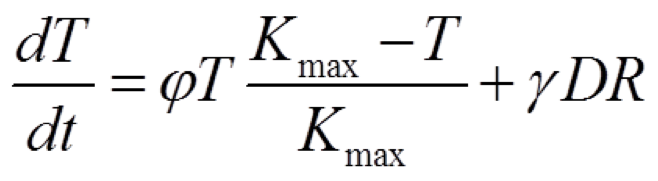The population dynamics for each strain are modeled based on logistic growth and are affected by conjugation. Conjugation, which is the term γDR, negatively affects the recipient cell population while positively contributing to the transconjugant cell population. The engineered donor cell remains unaffected by conjugation.

The parameters for these equations are shown below:

D – number of engineered donor cells
R – number of recipient cells
T – number of transconjugant cells
ϕ – maximum growth rate
Kmax – carrying capacity
γ – conjugation rate